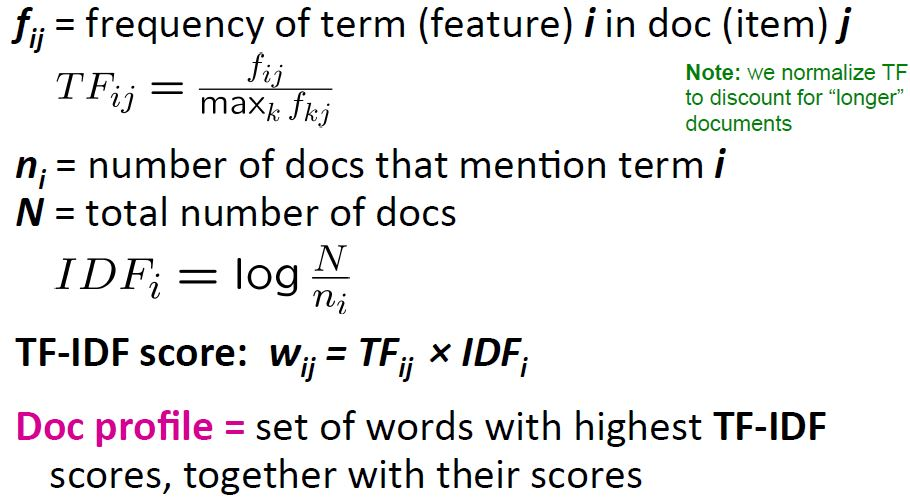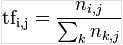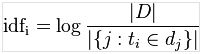# 文本特征提取

## 词袋（Bag of Words）表征

• 标记（tokenizing）文本以及为每一个可能的标记（token）分配的一个整型ID ，例如用白空格和标点符号作为标记的分割符（中文的话涉及到分词的问题）
• 计数（counting）标记在每个文本中的出现频率
• 正态化(nomalizating) 降低在大多数样本/文档中都出现的标记的权重

## 通用向量使用

CountVectorizer在一个类中实现了标记和计数：

from sklearn.feature_extraction.text import CountVectorizer


vectorizer = CountVectorizer(min_df=1)
vectorizer

CountVectorizer(analyzer=...'word', binary=False, charset=None,
charset_error=None, decode_error=...'strict',
dtype=<... 'numpy.int64'>, encoding=...'utf-8', input=...'content',
lowercase=True, max_df=1.0, max_features=None, min_df=1,
ngram_range=(1, 1), preprocessor=None, stop_words=None,
strip_accents=None, token_pattern=...'(?u)\\b\\w\\w+\\b',
tokenizer=None, vocabulary=None)


corpus = [
'This is the first document.',
'This is the second second document.',
'And the third one.',
'Is this the first document?',
]
X = vectorizer.fit_transform(corpus)
X

<4x9 sparse matrix of type '<... 'numpy.int64'>'
with 19 stored elements in Compressed Sparse Column format>


analyze = vectorizer.build_analyzer()
analyze("This is a text document to analyze.") == (
['this', 'is', 'text', 'document', 'to', 'analyze'])

True


vectorizer.get_feature_names() == (
['and', 'document', 'first', 'is', 'one',
'second', 'the', 'third', 'this'])

True

X.toarray()
array([[0, 1, 1, 1, 0, 0, 1, 0, 1],
[0, 1, 0, 1, 0, 2, 1, 0, 1],
[1, 0, 0, 0, 1, 0, 1, 1, 0],
[0, 1, 1, 1, 0, 0, 1, 0, 1]]...)


vectorizer.vocabulary_.get('document')

1


vectorizer.transform(['Something completely new.']).toarray()

array([[0, 0, 0, 0, 0, 0, 0, 0, 0]]...)


bigram_vectorizer = CountVectorizer(ngram_range=(1, 2),
token_pattern=r'\b\w+\b', min_df=1)
analyze = bigram_vectorizer.build_analyzer()
analyze('Bi-grams are cool!') == (
['bi', 'grams', 'are', 'cool', 'bi grams', 'grams are', 'are cool'])

True


X_2 = bigram_vectorizer.fit_transform(corpus).toarray()
X_2
...
array([[0, 0, 1, 1, 1, 1, 1, 0, 0, 0, 0, 0, 1, 1, 0, 0, 0, 0, 1, 1, 0],
[0, 0, 1, 0, 0, 1, 1, 0, 0, 2, 1, 1, 1, 0, 1, 0, 0, 0, 1, 1, 0],
[1, 1, 0, 0, 0, 0, 0, 0, 1, 0, 0, 0, 1, 0, 0, 1, 1, 1, 0, 0, 0],
[0, 0, 1, 1, 1, 1, 0, 1, 0, 0, 0, 0, 1, 1, 0, 0, 0, 0, 1, 0, 1]]...)


feature_index = bigram_vectorizer.vocabulary_.get('is this')
X_2[:, feature_index]

array([0, 0, 0, 1]...)


## TF-IDF(Term frequency * Inverse Doc Frequency)词权重IDF对于common words值小，而对rare words值大。

[ 百度百科tf-idf]

### sklearn实现TF-IDF

text.TfidfTransformer类实现了这种正态化：

from sklearn.feature_extraction.text import TfidfTransformer
transformer = TfidfTransformer()
transformer

TfidfTransformer(norm=...'l2', smooth_idf=True, sublinear_tf=False,
use_idf=True)


counts = [[3, 0, 1],
[2, 0, 0],
[3, 0, 0],
[4, 0, 0],
[3, 2, 0],
[3, 0, 2]]
tfidf = transformer.fit_transform(counts)
tfidf

<6x3 sparse matrix of type '<... 'numpy.float64'>'
with 9 stored elements in Compressed Sparse Row format>

tfidf.toarray()

array([[ 0.85...,  0.  ...,  0.52...],
[ 1.  ...,  0.  ...,  0.  ...],
[ 1.  ...,  0.  ...,  0.  ...],
[ 1.  ...,  0.  ...,  0.  ...],
[ 0.55...,  0.83...,  0.  ...],
[ 0.63...,  0.  ...,  0.77...]])


transformer.idf_

array([ 1. ...,  2.25...,  1.84...])


from sklearn.feature_extraction.text import TfidfVectorizer
vectorizer = TfidfVectorizer(min_df=1)
vectorizer.fit_transform(corpus)
...
<4x9 sparse matrix of type '<... 'numpy.float64'>'
with 19 stored elements in Compressed Sparse Row format>


[皮皮blog]

## 解码文本文件

• 找到文本的实际编码。文件可能包含一个文件头告诉你它的编码，或者根据文本的来自哪里你可以猜测一些标准的编码。
• 用UNIX命令file，你可以找到它是什么编码。Python的chardet模块有一个叫做chardetect.py的脚本，这个脚本会猜测具体的编码，尽管你不能期望它的猜测就是正确的。
• 你可以忽略错误，试一下UTF-8。你可以用bytes.decode(errors='replace')来解码字节字符串，用一个无意义的字符来替换所有解码错误，或者在向量器中设置decode_error='replace'。这可能特征的有用性。
• 真实的文本可能来自多种来源使用多种编码，甚至可能凌乱的用错误的编码解码。在网络上的文本检索过程中这非常常见。Python的包ftfy可以自动挑选出几类解码错误，因此，你可以尝试尝试将位置文档解码为latin-1，然后用ftfy来修复错误。
• 如果文本是一坨乱七八糟的东西（20个新闻组的数据集就是这样），很难简单的分类出编码，你可以回滚到单字符编码，比如latin-1。一些文本的显示可能不正确，但是，至少相同的字节序列都会代表相同的特征。

## 词袋表征的局限

N-grams来帮忙！与构建简单的一组unigrams相比，人们更倾向于构建一组bigrams（n=2），计数一组成对连续出现的词的频率。

ngram_vectorizer = CountVectorizer(analyzer='char_wb', ngram_range=(2, 2), min_df=1)
counts = ngram_vectorizer.fit_transform(['words', 'wprds'])
ngram_vectorizer.get_feature_names() == (
[' w', 'ds', 'or', 'pr', 'rd', 's ', 'wo', 'wp'])

True

counts.toarray().astype(int)

array([[1, 1, 1, 0, 1, 1, 1, 0],
[1, 1, 0, 1, 1, 1, 0, 1]])


ngram_vectorizer = CountVectorizer(analyzer='char_wb', ngram_range=(5, 5), min_df=1)
ngram_vectorizer.fit_transform(['jumpy fox'])

<1x4 sparse matrix of type '<... 'numpy.int64'>'
with 4 stored elements in Compressed Sparse Column format>

ngram_vectorizer.get_feature_names() == (
[' fox ', ' jump', 'jumpy', 'umpy '])

True

ngram_vectorizer = CountVectorizer(analyzer='char', ngram_range=(5, 5), min_df=1)
ngram_vectorizer.fit_transform(['jumpy fox'])

<1x5 sparse matrix of type '<... 'numpy.int64'>'
with 5 stored elements in Compressed Sparse Column format>

ngram_vectorizer.get_feature_names() == (
['jumpy', 'mpy f', 'py fo', 'umpy ', 'y fox'])

True


## 用哈希技巧向量化大文本向量

from sklearn.feature_extraction.text import HashingVectorizer
hv = HashingVectorizer(n_features=10)
hv.transform(corpus)
...
<4x10 sparse matrix of type '<... 'numpy.float64'>'
with 16 stored elements in Compressed Sparse Row format>


hv = HashingVectorizer()
hv.transform(corpus)
...
<4x1048576 sparse matrix of type '<... 'numpy.float64'>'
with 19 stored elements in Compressed Sparse Row format>


HashingVectorizer也有以下的局限：

• 不能反转模型（没有inverse_transform方法），也无法访问原始的字符串表征，因为，进行mapping的哈希方法是单向本性。
• 没有提供了IDF权重，因为这需要在模型中引入状态。如果需要的话，可以在管道中添加TfidfTransformer。

## 进行HashingVectorizer的核外扩展

http://cloga.info/2014/01/19/sklearn_text_feature_extraction/

07-2611607-218935
01-051万+
08-281983
03-271万+
09-307161
12-19203
10-244790
09-188463